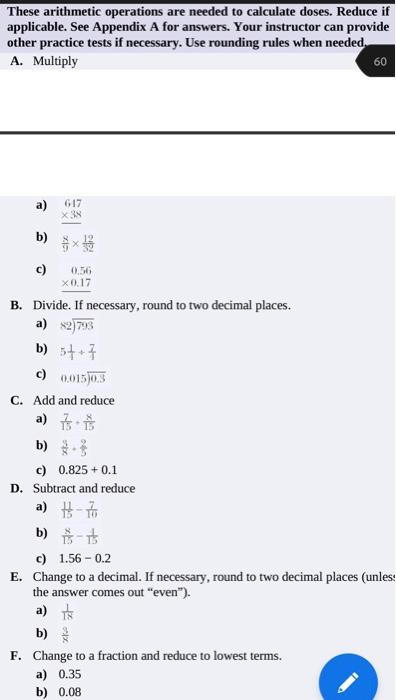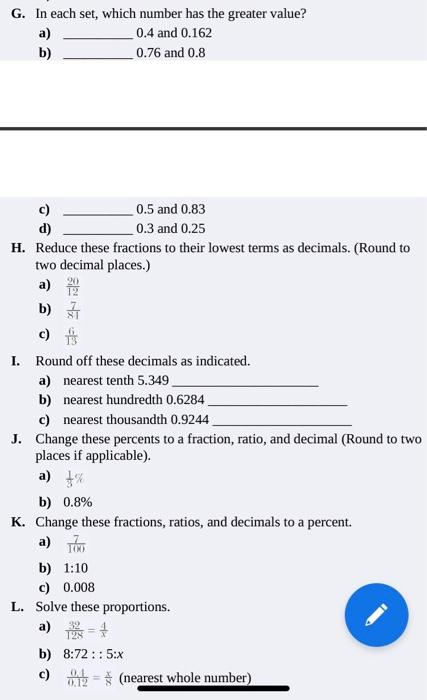# (Solved): Please help me D, E, F, G, H, I, J, K, L.  These arithmetic operations are needed to calculate ...These arithmetic operations are needed to calculate doses. Reduce if applicable. See Appendix A for answers. Your instructor can provide other practice tests if necessary. Use rounding rules when needed, A. Multiply 60 a) 617 b) $$\frac{8}{9} \times \frac{12}{32}$$ c) $$\begin{array}{r}0.56 \\ \times 0.17 \\ \hline\end{array}$$ B. Divide. If necessary, round to two decimal places. a) $$\times 2 \longdiv { 7 9 3 }$$ b) $$5 \frac{1}{1}+7$$ c) $$0 . 0 1 5 \longdiv { 0 . 3 }$$ C. Add and reduce a) $$\frac{7}{15}+\frac{8}{15}$$ b) $$\frac{3}{8}+\frac{2}{3}$$ c) $$0.825+0.1$$ D. Subtract and reduce a) $$\frac{11}{15}-\frac{7}{10}$$ b) $$\frac{8}{15}-\frac{1}{15}$$ c) $$1.56-0.2$$ E. Change to a decimal. If necessary, round to two decimal places (unless the answer comes out "even"). a) $$\frac{1}{18}$$ b) $$\frac{3}{8}$$ F. Change to a fraction and reduce to lowest terms. a) $$0.35$$ b) $$0.08$$ G. In each set, which number has the greater value? a) $$0.4$$ and $$0.162$$ b) $$0.76$$ and $$0.8$$ c) d) $$0.5$$ and $$0.83$$ $$0.3$$ and $$0.25$$ H. Reduce these fractions to their lowest terms as decimals. (Round to two decimal places.) a) $$\frac{20}{12}$$ b) $$\frac{7}{81}$$ c) $$\frac{6}{13}$$ I. Round off these decimals as indicated. a) nearest tenth $$5.349$$ b) nearest hundredth $$0.6284$$ c) nearest thousandth $$0.9244$$ J. Change these percents to a fraction, ratio, and decimal (Round to two places if applicable). a) $$\frac{1}{3} \%$$ b) $$0.8 \%$$ K. Change these fractions, ratios, and decimals to a percent. a) $$\frac{7}{1 \times 1}$$ b) $$1: 10$$ c) $$0.008$$ L. Solve these proportions. a) $$\frac{32}{125}=\frac{4}{x}$$ b) $$8: 72:: 5: x$$ c) $$\frac{0.1}{0.12}=\frac{x}{8}$$ (nearest whole number)

We have an Answer from Expert